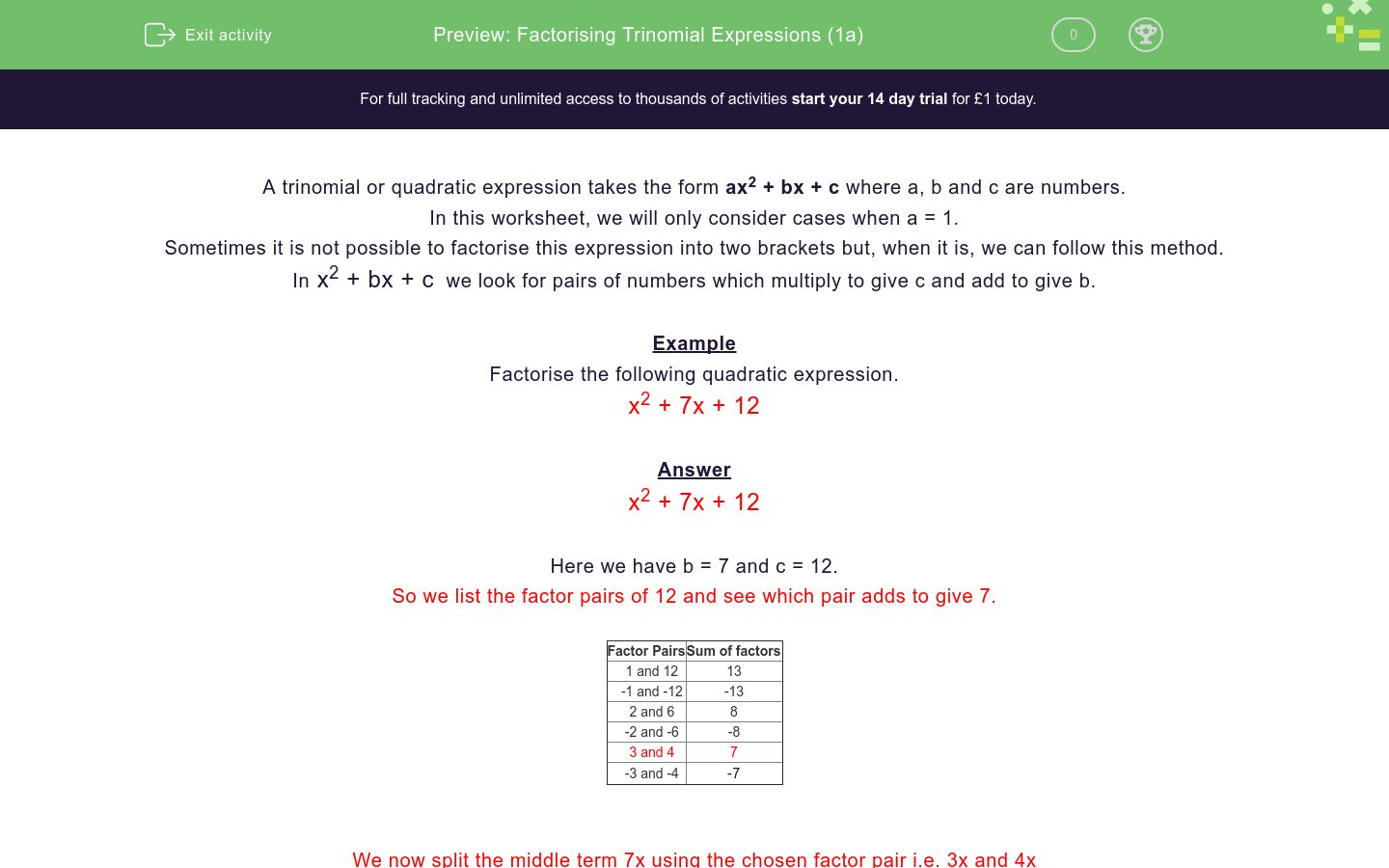# Factorising Trinomial Expressions (1a)

In this worksheet, students factorise simple trinomial expressions.Key stage:  KS 4

Curriculum topic:  Algebra

Curriculum subtopic:  Simplify/Manipulate Algebraic Expressions/Fractions

Difficulty level:### QUESTION 1 of 10

A trinomial or quadratic expression takes the form ax2 + bx + c where a, b and c are numbers.

In this worksheet, we will only consider cases when a = 1.

Sometimes it is not possible to factorise this expression into two brackets but, when it is, we can follow this method.

In x2 + bx + c  we look for pairs of numbers which multiply to give c and add to give b.

Example

x2 + 7x + 12

x2 + 7x + 12

Here we have b = 7 and c = 12.

So we list the factor pairs of 12 and see which pair adds to give 7.

Factor Pairs Sum of factors
1 and 12 13
-1 and -12 -13
2 and 6 8
-2 and -6 -8
3 and 4 7
-3 and -4

-7

We now split the middle term 7x using the chosen factor pair i.e. 3x and 4x

x2 + 3x + 4x +12

Consider the expression in pairs

x2 + 3x + 4x + 12

Factorise each pair

x (x + 3) + 4 (x + 3)

Combine

(x + 4) (x + 3)

This is the full factorisation

(x + 4) (x + 3)

x2 + 5x + 6

(x + 2) (x + 6)

(x + 1) (x + 6)

(x + 3) (x + 2)

(x + 2) (x + 4)

x2 + 8x + 12

(x + 2) (x + 6)

(x + 1) (x + 6)

(x + 3) (x + 2)

(x + 2) (x + 4)

x2 + 6x + 8

(x + 2) (x + 6)

(x + 1) (x + 6)

(x + 3) (x + 2)

(x + 2) (x + 4)

x2 + 7x + 6

(x + 2) (x + 6)

(x + 1) (x + 6)

(x + 3) (x + 2)

(x + 2) (x + 4)

x2 + 10x + 9

(x + 4) (x + 4)

(x + 2) (x + 8)

(x + 1) (x + 9)

(x + 3) (x + 3)

x2 + 6x + 9

(x + 4) (x + 4)

(x + 2) (x + 8)

(x + 1) (x + 9)

(x + 3) (x + 3)

x2 + 10x + 16

(x + 4) (x + 4)

(x + 2) (x + 8)

(x + 1) (x + 9)

(x + 3) (x + 3)

x2 + 8x + 16

(x + 4) (x + 4)

(x + 2) (x + 8)

(x + 1) (x + 9)

(x + 3) (x + 3)

x2 + 20x + 36

(x + 9) (x + 4)

(x + 6) (x + 6)

(x + 12) (x + 3)

(x + 18) (x + 2)

x2 + 13x + 36

(x + 9) (x + 4)

(x + 6) (x + 6)

(x + 12) (x + 3)

(x + 18) (x + 2)

• Question 1

x2 + 5x + 6

(x + 3) (x + 2)
• Question 2

x2 + 8x + 12

(x + 2) (x + 6)
• Question 3

x2 + 6x + 8

(x + 2) (x + 4)
• Question 4

x2 + 7x + 6

(x + 1) (x + 6)
• Question 5

x2 + 10x + 9

(x + 1) (x + 9)
• Question 6

x2 + 6x + 9

(x + 3) (x + 3)
• Question 7

x2 + 10x + 16

(x + 2) (x + 8)
• Question 8

x2 + 8x + 16

(x + 4) (x + 4)
• Question 9

x2 + 20x + 36

(x + 18) (x + 2)
• Question 10

x2 + 13x + 36